Back

## Electricity

### In text 12.1 Page: 200

1. What does an electric circuit mean?

Solution:

A continuous closed path made of electric components through which an electric current flows is known as an electric circuit. A simple circuit consists of the following components:

(a) Conductors

(b) Cell

(c) Switch

2. Define the unit of current.

Solution:

The unit of current is ampere. Ampere is defined by the flow of one coulomb of charge per second.

3. Calculate the number of electrons constituting one coulomb of charge.

Solution:

The value of the charge of an electron is 1.6 × 10-19 C.

According to charge quantization,

Q = nqewhere n is the number of electrons and qe is the charge of an electron.

Substituting the values in the above equation, the number of electrons in a coulomb of charge can be calculated as follows: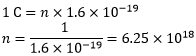Therefore, the number of electrons constituting one coulomb of charge is 6. 25 × 1018.

In text 12.2 Page: 202

1. Name a device that helps to maintain a potential difference across a conductor.

Solution:

Battery consisting of one or more electric cells is one of the devices that help to maintain a potential difference across a conductor.

2. What is meant by saying that the potential difference between two points is 1 V?

Solution:

When 1 J of work is done to move a charge of 1 C from one point to another, it is said that the potential difference between two points is 1 V.

3. How much energy is given to each coulomb of charge passing through a 6 V battery?

Solution:

We know that the potential difference between two points is given by the equation,

V = W/Q, where,

W is the work done in moving the charge from one point to another

Q is the charge

From the above equation, we can find the energy given to each coulomb as follows:

W = V × Q

Substituting the values in the equation, we get

W = 6V × 1C = 6 J

Hence, 6 J of energy is given to each coulomb of charge passing through a 6 V of battery.

## In text 12.5 Page: 209

1. On what factors does the resistance of a conductor depend?

Solution:

The resistance of the conductor depends on the following factors:

a. Temperature of the conductor

b. Cross-sectional area of the conductor

c. Length of the conductor

d. Nature of the material of the conductor

2. Will current flow more easily through a thick wire or a thin wire of the same material, when connected to the same source? Why?

Solution:

Resistance is given by the equation,

R = ρ l/A

where,

ρ is the resistivity of the material of the wire,

l is the length of the wire

A is the area of the cross-section of the wire.

From the equation, it is evident that the area of the cross-section of wire is inversely proportional to the resistance. Therefore, thinner the wire, more the resistance and vice versa. Hence, current flows more easily through a thick wire than a thin wire.

3. Let the resistance of an electrical component remain constant while the potential difference across the two ends of the component decreases to half of its former value. What change will occur in the current through it?

Solution:

The change in the current flowing through the electrical component can be determined by Ohm’s Law.

According to Ohm’s Law, the current is given by

I = V/R

Now, the potential difference is reduced to half keeping the resistance constant,

Let the new voltage be V’ = V/2

Let the new resistance be R’ = R and the new amount of current be I’.

The change in the current can be determined using Ohm’s law as follows: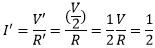Therefore, the current flowing the electrical component is reduced by half.

4. Why are coils of electric toasters and electric irons made of an alloy rather than a pure metal?

Solution:

The melting point of an alloy is much higher than a pure metal because of its high resistivity. At high temperatures, alloys do not melt readily. Therefore, alloys are used in heating appliances such as electric toasters and electric irons.

5. Use the data in the table given below and answer the following questions.

 Material Resistivity Conductors Silver 1.60 × 10–8 Copper 1.62 × 10–8 Aluminium 2.63 × 10–8 Tungsten 5.20 × 10–8 Nickel 6.84 × 10–8 Iron 10.0 × 10–8 Chromium 12.9 × 10–8 Mercury 94.0 × 10–8 Manganese 1.84 × 10–6 Alloys Constantan 49 × 10–6 Manganin 44 × 10–6 Nichrome 100 × 10–6 Insulators Glass 1010 – 1014 Hard rubber 1013 – 1016 Ebonite 1015 – 1017 Diamond 1012 – 1013 Paper (dry) 1012

a. Which among iron and mercury is a better conductor?

b. Which material is the best conductor?

Solution:

a. Iron is a better conductor than mercury because the resistivity of mercury is more than the resistivity of iron.

b. Among all the materials listed in the table, silver is the best conductor because the resistivity of silver is lowest among all, i.e., 1.60 × 10–8.

## In text 12.6 Page: 213

1. Draw a schematic diagram of a circuit consisting of a battery of three cells of 2 V each, a 5 Ω resistor, an 8 Ω resistor, and a 12 Ω resistor, and a plug key, all connected in series.

Solution:

A battery of three cells of 2 V each equals to battery of potential 6 V. The circuit diagram below shows three resistors of resistance 12 Ω, 8 Ω and 5 Ω connected in series along with a battery of potential 6 V.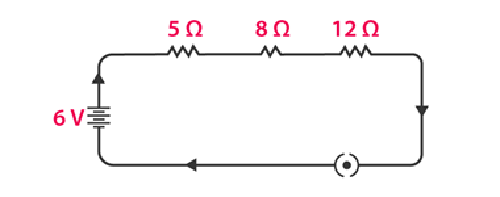2. Redraw the circuit of Question 1, putting in an ammeter to measure the current through the resistors and a voltmeter to measure the potential difference across the 12 Ω resistor. What would be the readings in the ammeter and the voltmeter?

Solution:

An ammeter should always be connected in series with resistors while the voltmeter should be connected in parallel to the resistor to measure the potential difference as shown in the figure below.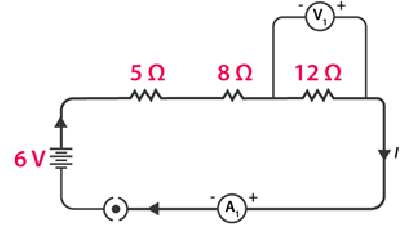Using Ohm’s Law, we can obtain the reading of the ammeter and the voltmeter.

The total resistance of the circuit is 5 Ω + 8 Ω +12 Ω = 25 Ω.

We know that the potential difference of the circuit is 6 V, hence the current flowing through the circuit or the resistors can be calculated as follows:

I = V/R = 6/25 = 0.24A

Let the potential difference across the 12 Ω resistor be V1.

From the obtained current V1 can be calculated as follows:

V1 = 0.24A × 12 Ω = 2.88 V

Therefore, the ammeter reading will be 0.24 A and the voltmeter reading be 2.88 V.

## In text 12.6.2 Page: 216

1. Judge the equivalent resistance when the following are connected in parallel – (a) 1 Ω and 106 Ω, (b) 1 Ω, 103 Ω, and 106 Ω.

Solution:

(a) When 1 Ω and 106 are connected in parallel, the equivalent resistance is given by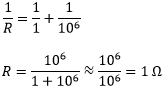Therefore, the equivalent resistance is 1 Ω.

(b) When 1 Ω, 103 Ω, and 106 Ω are connected in parallel, the equivalent resistance is given by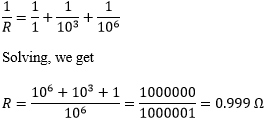Therefore, the equivalent resistance is 0.999 Ω.

2. An electric lamp of 100 Ω, a toaster of resistance 50 Ω, and a water filter of resistance 500 Ω are connected in parallel to a 220 V source. What is the resistance of an electric iron connected to the same source that takes as much current as all three appliances, and what is the current through it?

Solution:

The electric lamp, the toaster and the water filter connected in parallel to a 220 V source can be shown as using a circuit diagram as follows: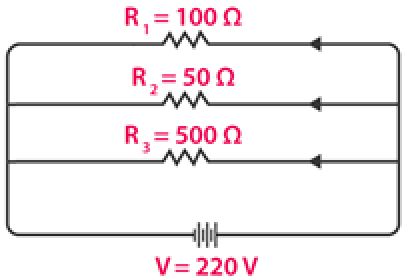The equivalent resistance of the resistors can be calculated as follows: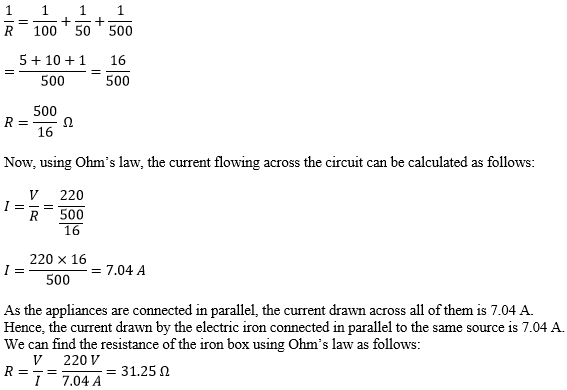The resistance of the electric iron box is 31.25 Ω.

3. What are the advantages of connecting electrical devices in parallel with the battery instead of connecting them in series?

Solution:

When the electrical devices are connected in parallel there is no division of voltage among the appliances. The potential difference across the devices is equal to supply voltage. Parallel connection of devices also reduces the effective resistance of the circuit.

4. How can three resistors of resistances 2 Ω, 3 Ω, and 6 Ω be connected to give a total resistance of (a) 4 Ω, (b) 1 Ω?

Solution:

(a) The circuit diagram below shows the connection of three resistors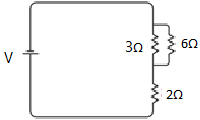From the circuit above, it is understood that 3 Ω and 6 Ω are connected in parallel. Hence, their equivalent resistance is given by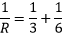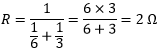The equivalent resistor 2 Ω is in series with the 2 Ω resistor. Now the equivalent resistance can be calculated as follows:

Req= 2 Ω +2 Ω = 4 Ω

Hence, the total resistance of the circuit is 4 Ω.

(b) The circuit diagram below, shows the connection of three resistors.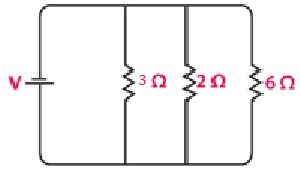From the circuit, it is understood that all the resistors are connected in parallel. Therefore, their equivalent resistance can be calculated as follows: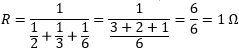The total resistance of the circuit is 1 Ω.

5. What is (a) the highest, (b) the lowest total resistance that can be secured by combinations of four coils of resistance 4 Ω, 8 Ω, 12 Ω, 24 Ω?

Solution:

(a) If the four resistors are connected in series, their total resistance will be the sum of their individual resistances and it will be the highest. The total equivalent resistance of the resistors connected in series will be 4 Ω + 8 Ω + 12 Ω + 24 Ω = 48 Ω.

(b) If the resistors are connected in parallel, then their equivalent resistances will be the lowest.

Their equivalent resistance connected in parallel is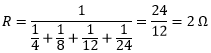Hence, the lowest total resistance is 2 Ω.

## In text 12.7 Page: 218

1. Why does the cord of an electric heater not glow while the heating element does?

Solution:

The heating element of an electric heater is made of an alloy which has a high resistance. When the current flows through the heating element, the heating element becomes too hot and glows red. The cord is usually made of copper or aluminum which has low resistance. Hence the cord doesn’t glow.

2. Compute the heat generated while transferring 96000 coulomb of charge in one hour through a potential difference of 50 V.

Solution:

The heat generated can be computed by Joule’s law as follows:

H = VIt

where,

V is the voltage, V = 50 V

I is the current

t is the time in seconds, 1 hour = 3600 seconds

The amount of current can be calculated as follows: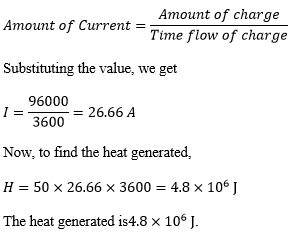2. An electric iron of resistance 20 Ω takes a current of 5 A. Calculate the heat developed in 30 s.

Solution:

The amount of heat generated can be calculated using the Joule’s law of heating, which is given by the equation

H = VIt

Substituting the values in the above equation, we get,

H = 100 × 5 × 30 = 1.5 × 104 J

The amount of heat developed by the electric iron in 30 s is 1.5 × 104 J.

## In text 12.8 Page: 220

1. What determines the rate at which energy is delivered by a current?

Solution:

Electric power is the rate of consumption of electrical energy by electric appliances. Hence, the rate at which energy is delivered by a current is the power of the appliance.

2. An electric motor takes 5 A from a 220 V line. Determine the power of the motor and the energy consumed in 2 h.

Solution:

The power of the motor can be calculated by the equation,

P = VI

Substituting the values in the above equation, we get

P = 220 V × 5 A = 1100 W

The energy consumed by the motor can be calculated using the equation,

E = P × T

Substituting the values in the above equation, we get

P = 1100 W × 7200 = 7.92 × 106 J

The power of the motor is 1100 W and the energy consumed by the motor in 2 hours is 7.92 × 106 J.

## Exercises Page: 221

1. A piece of wire of resistance R is cut into five equal parts. These parts are then connected in parallel. If the equivalent resistance of this combination is R′, then the ratio R/R′ is _____.

(a) 1/25

(b) 1/5

(c) 5

(d) 25

Solution:

Explanation:

The resistance is cut into five equal parts, which means that the resistance of each part is R/5.

We know that each part is connected to each other in parallel, hence the equivalent resistance can be calculated as follows: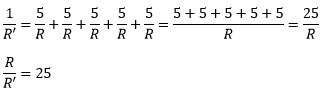The ratio of R/R′ is 25.

2. Which of the following does not represent electrical power in a circuit?

(a) I2R

(b) IR2

(c) VI

(d) V2/R

Solution:

Explanation:

Electrical power is given by the expression P = VI. (1)

According to Ohm’s law,

V = IR

Substituting the value of V in (1), we get

P = (IR) × I

P = I2R

Similarly, from Ohm’s law,

I = V/R

Substituting the value of I in (1), we get

P = V × V/R = V2/R

From this, it is clear that the equation IR2 does not represent electrical power in a circuit.

3. An electric bulb is rated 220 V and 100 W. When it is operated on 110 V, the power consumed will be _____.

(a) 100 W

(b) 75 W

(c) 50 W

(d) 25 W

Solution:

Explanation:

The energy consumed by the appliance is given by the expression

P = VI = V2/R

The resistance of the light bulb can be calculated as follows:

R = V2/P

Substituting the values, we get

R = (220)2/100 = 484 Ω

Even if the supply voltage is reduced, the resistance remains the same. Hence, the power consumed can be calculated as follows:

P = V2/R

Substituting the value, we get

P = (110)2 V/484 Ω = 25 W

Therefore, the power consumed when the electric bulb operates at 110 V is 25 W.

4. Two conducting wires of the same material and of equal lengths and equal diameters are first connected in series and then parallel in a circuit across the same potential difference. The ratio of heat produced in series and parallel combinations would be _____.

(a) 1:2

(b) 2:1

(c) 1:4

(d) 4:1

Solution:

Let Rs and Rp be the equivalent resistance of the wires when connected in series and parallel respectively.

For the same potential difference V, the ratio of the heat produced in the circuit is given by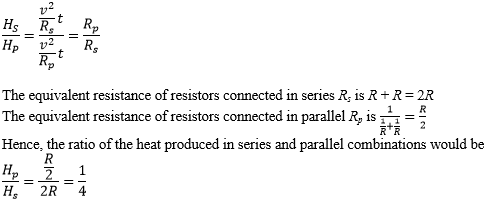Hence, the ratio of the heat produced is 1:4.

5. How is a voltmeter connected in the circuit to measure the potential difference between two points?

Solution:

To measure the voltage between any two points, the voltmeter should be connected in parallel between the two points.

6. A copper wire has diameter 0.5 mm and resistivity of 1.6 × 10–8 Ω m. What will be the length of this wire to make its resistance 10 Ω? How much does the resistance change if the diameter is doubled?

Solution:

The resistance of the copper wire of length in meters and area of cross-section mis given by the formula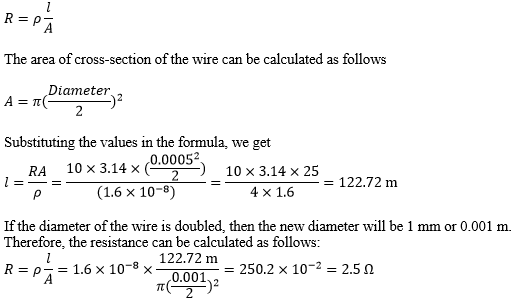The length of the wire is 122.72 m and the new resistance is 2.5 Ω.

7. The values of current I flowing in a given resistor for the corresponding values of potential difference V across the resistor are given below –

 I (Ampere) 0.5 1 2 3 4 V (Volts) 1.6 3.4 6.7 10.2 13.2

Plot a graph between V and I and calculate the resistance of that resistor.

Solution:

The plot between voltage and current is known as IV characteristic. The current is plotted in the y-axis while the voltage is plotted in the x-axis. The different values of current for different values of voltage are given in the table. The I V characteristics for the given resistor is shown below.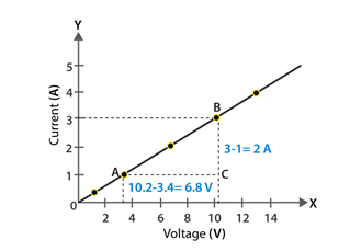The slope of the line gives the value of resistance.

The slope can be calculated as follows:

Slope = 1/R = BC/AC = 2/6.8

To calculate R,

R = 6.8/2 = 3.4 Ω

The resistance of the resistor is 3.4 Ω.

8. When a 12 V battery is connected across an unknown resistor, there is a current of 2.5 mA in the circuit. Find the value of the resistance of the resistor

Solution:

The value of the resistor can be calculated using Ohm’s Law as follows: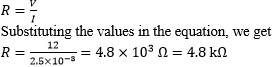9. A battery of 9 V is connected in series with resistors of 0.2 Ω, 0.3 Ω, 0.4 Ω, 0.5 Ω and 12 Ω, respectively. How much current would flow through the 12 Ω resistor?

Solution:

In series connection, there is no division of current. The current flowing across all the resistors is the same.

To calculate the amount of current flowing across the resistors, we use Ohm’s law.

But first, let us find out the equivalent resistance as follows:

R = 0.2 Ω + 0.3 Ω + 0.4 Ω + 0.5 Ω + 12 Ω = 13.4 Ω

Now, using Ohm’s law,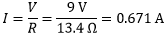The current flowing across the 12 Ω is 0.671 A.

10. How many 176 Ω resistors (in parallel) are required to carry 5 A on a 220 V line?

Solution:

Let us consider the number of resistors required as ‘x.’

The equivalent resistance of the parallel combination of resistor R is given by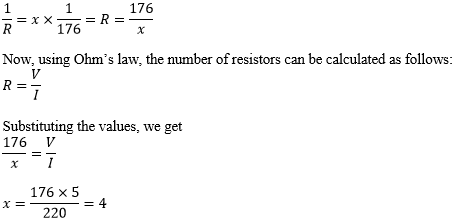The number of resistors required is 4.

11. Show how you would connect three resistors, each of resistance 6 Ω, so that the combination has a resistance of (i) 9 Ω, (ii) 4 Ω.

Solution:

If we connect all the three resistors in series, their equivalent resistor would 6 Ω + 6 Ω + 6 Ω =18 Ω, which is not the desired value. Similarly, if we connect all the three resistors in parallel, their equivalent resistor would be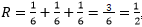which is again not the desired value.

We can obtain the desired value by connecting any two of the resistors in either series or parallel.

Case (i)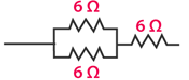If two resistors are connected in parallel, then their equivalent resistance is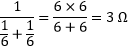The third resistor is in series, hence the equivalent resistance is calculated as follows:

R = 6 Ω + 3 Ω = 9 Ω

Case (ii)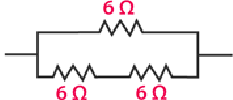When two resistors are connected in series, their equivalent resistance is given by

R = 6 Ω + 6 Ω = 12 Ω

The third resistor is connected in parallel with 12 Ω. Hence the equivalent resistance is calculated as follows: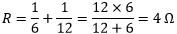12. Several electric bulbs designed to be used on a 220 V electric supply line, are rated 10 W. How many lamps can be connected in parallel with each other across the two wires of 220 V line if the maximum allowable current is 5 A?

Solution:

The resistance of the bulb can be calculated using the expression

P1 = V2/R1

R1 = V2/P1

Substituting the values, we get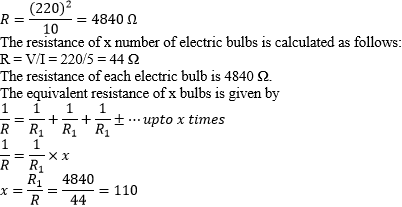Hence, 110 lamps can be connected in parallel.

13. A hot plate of an electric oven connected to a 220 V line has two resistance coils A and B, each of 24 Ω resistance, which may be used separately, in series, or in parallel. What are the currents in the three cases?

Solution:

Case (i) When coils are used separately

Using Ohm’s law, we can find the current flowing through each coil as follows: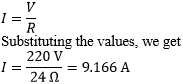9.166 A of current flows through each resistor when they are used separately.

Case (ii) When coils connected in series

The total resistance in the series circuit is 24 Ω + 24 Ω = 48 Ω

The current flowing through the series circuit is calculated as follows: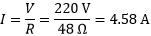Therefore, a current of 4.58 A flows through the series circuit.

Case (iii) When coils connected in parallel

When the coils are connected in parallel, the equivalent resistance is calculated as follows: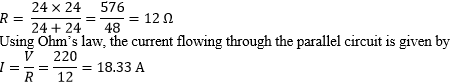The current in the parallel circuit is 18.33 A.

14. Compare the power used in the 2 Ω resistor in each of the following circuits: (i) a 6 V battery in series with 1 Ω and 2 Ω resistors, and (ii) a 4 V battery in parallel with 12 Ω and 2 Ω resistors.

Solution:

(i) The potential difference is 6 V and the resistors 1 Ω and 2 Ω are connected in series, hence their equivalent resistance is given by 1 Ω + 2 Ω = 3 Ω. The current in the circuit can be calculated using the Ohm’s law as follows: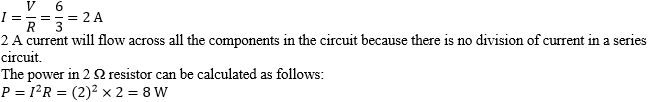Therefore, the power consumed by the 2 Ω is 8 W.

(ii) When 12 Ω and 2 Ω resistors are connected in parallel, the voltage across the resistors remains the same. Knowing that the voltage across 2 Ω resistor is 4 V, we can calculate the power consumed by the resistor as follows: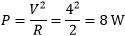The power consumed by the 2 Ω resistor is 8 W.

15. Two lamps, one rated 100 W at 220 V, and the other 60 W at 220 V, are connected in parallel to electric mains supply. What current is drawn from the line if the supply voltage is 220 V?

Solution:

Since both the bulbs are connected in parallel, the voltage across each of them will be the same.

Current drawn by the bulb of rating 100 W can be calculated as follows:

P = V × I

I = P/V

Substituting the values in the equation, we get

I = 100 W/220 V = 100/220 A

Similarly, the current drawn by the bulb of rating 60 W can be calculated as follows:

I = 60 W/220 V = 60/220 A

Therefore, the current drawn from the line is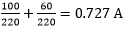16. Which uses more energy, a 250 W TV set in 1 hr, or a 1200 W toaster in 10 minutes?

Solution:

The energy consumed by electrical appliances is given by the equation

Pt, where P is the power of the appliance and t is the time

Using this formula, the energy consumed by a TV of power ration 250 W, can be calculated as follows:

H = 250 W × 3600 seconds = 9 × 105 J

Similarly, the energy consumed by a toaster of power rating 1200 W is

H = 1200 W × 600 s = 7.2 × 105 J

From the calculations, it can be said that the energy consumed by the TV is greater than the toaster.

17. An electric heater of resistance 8 Ω draws 15 A from the service mains 2 hours. Calculate the rate at which heat is developed in the heater.

Solution:

The rate at which the heat develops in the heater can be calculated using the following formula

P = I2 R

Substituting the values in the equation, we get

P = (15A) 2 × 8 Ω = 1800 J/s

The electric heater produces heat at the rate of 1800 J/s

18. Explain the following.

a. Why is the tungsten used almost exclusively for filament of electric lamps?

b. Why are the conductors of electric heating devices, such as bread-toasters and electric irons, made of an alloy rather than a pure metal?

c. Why is the series arrangement not used for domestic circuits?

d. How does the resistance of a wire vary with its area of cross-section?

e. Why copper and aluminium wires are usually employed for electricity transmission?

Solution:

a. The resistivity and melting point of tungsten is very high. Due to this property, it doesn’t burn readily when heated. Electric lamps operate at high temperature. Hence, tungsten is a choice of metal for the filament of electric lamps.

b. The conductors of electric heating devices are alloys because of their high resistivity. Due to its high resistivity it produces large amount of heat.

c. The voltage is divided in series circuit as result each component in the circuit receives a small voltage because of which the amount of current decreases and the device gets hot and does not work properly. This is the reason why series circuits are not used in domestic circuits.

d. Resistance is inversely proportional to the area of cross section. When the area of cross section increases the resistance decreases and vice versa.

e. Copper and aluminium are good conductors of electricity and have low resistivity because of which they are usually employed for electricity transmission.

### Comprehensive List Of Study Materials